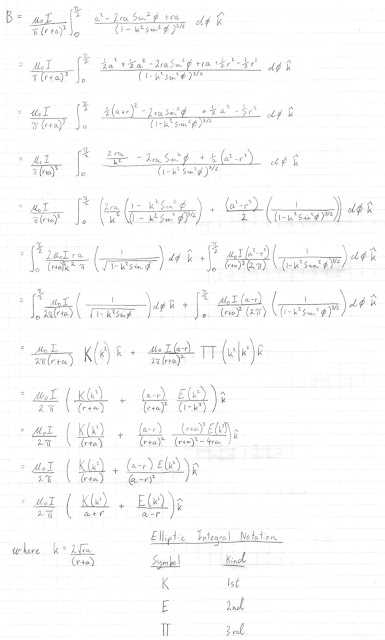## Wednesday, December 2, 2015

### I hope you find this blog enjoyable and helpful. Support it with a small donation.

Well, this one is a failure (for now).  In the comments on my post about the "Off Axis Magnetic Field of a Circular Current Loop" I was asked why I'd chosen to solve the problem the way I did and why I didn't calculate the Magnetic field using the more familiar version of the Biot Savart law.  I don't know.  I just happened to pick this method and it worked.  I was also asked about calculating the in plane magnetic field of a circular current loop and my response was use the 3 dimensional solution and set z to 0.

I did want to try and calculate it just using the Biot Savart law.  After all, the field shouldn't be that hard to work out.  Due to the geometry of the problem there can only be a magnetic field perpendicular to the plane of the current loop when z=0, and due to rotational symmetry you only need to calculate the magnetic filed along the x axis for example and then revolve it to generate the entire field.  Easy, except I just can't get it.  I'll show my attempt below.Magnetic field of a current loop derived from Biot Savart

That's where I hit a wall.  I've made some progress, but I just can't get the equation into a form that makes the use of elliptic integrals easy.  I took the vector cross product of the infinitesimal current element and the vector pointing from the current element to the point that the field is to be calculated at and divided that by the distance between these points squared.  This is then integrated around the current loop.Geometry of the situation

I've performed numerical integration using maxima at various steps of my calculation to make sure I haven't made any mistakes.  Although it's no guarantee I'm right, all the answers match.Current Loop In Plane Field Working

Looking at the equation below from my original blog post you can see the equation I'm aiming for if you set z to equal 0.  Plugging numbers into this also yields an answer equal to what my equation above gave.Desired Equation

I think this is one of those cases where it's all a mater of finding the right identity or change of variable.  I might let my subconscious work on it for a while and come back to it refreshed.

Update - 4 December 2015

I solved it.  It was relatively easy in the end. I just needed to take a break and look at it differently.In plane magnetic field of a circular current loop

### Support me and the blog with a small donation. I appreciate each one.

1.Good job Grant and thanks. By the way, how would you proceed with calculating the elliptic integrals from now on?

1.Unfortunately there's no easy way to do this. On the Wikipedia page for elliptic integrals you can see that they can be expressed as an infinite summation, however this isn't helpful. Before computers they were just looked up in a table similar to functions like sine and logarithms.

Most maths software will have functions to calculate them. Octave, Matlab, Maxima, Python, all have functions to do this.

2.Ok I understand, I appreciate your help.

2.This is brilliant, mate! Like you, I was unable to find this problem worked out anywhere on the internet. I set up the integrals for the in-plane version of this problem last year as an advanced problem for one of my better undergrad students in E&M. It is great to see you thinking like a physicist: checking the simple cases at the origin and checking that your more general solution in all of space reduces to this one in the plane of the loop. From the axial symmetry, we know that the only thing B_z can depend upon in the plane is r, and your solution agrees. It was also nice to see that you found the same solution from the pre-internet era. I haven't learned Maxima yet, but I plan to start by making a dual plot of E_2(k)/(a-r) and E_1(k)/(a+r) to demonstrate that inside the loop (r 0 and outside (R>a) B_z < 0, as the Right-hand Rule demands this circulation sense around the current, and show their expected crossover at r = a. Again, fine work!

1.Thanks for the kind words. Maxima is a bit hard initially, but after you understand its quirks it's pretty easy. If you have any more insights into this problem, I'd be glad to link to any blog or website you may have.